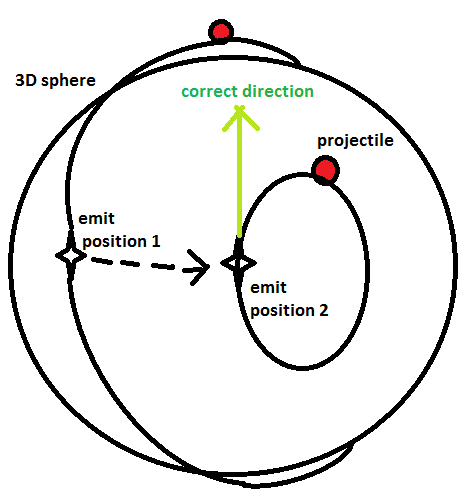# Rotate around sphere using Quaternions with full radius

Hi,

I am trying to shoot projectiles from the player’s position, who is on a surface of the sphere, and make the projectiles rotate around the sphere. Here’s (sloppy) 3D representation:Same stuff but from the side view:

The projectiles rotate correctly when initializing projectiles from emit position 1 a.k.a initial position.

When I translate player’s position and initialize a new projectile, it has smaller radius. The required direction should be the direction in which the green arrow is pointing at.

The code for rotation I use:

``````    public float projectileSpeed = 20f;     // Speed of the projectile
public GameObject projectile;           // Projectile's template
private GameObject aProjectile;         // Instantiated projectile
public Vector3 centerPosition;          // origin (0,0,0)

void Update() {
if (Input.GetButtonDown("Fire1"))
{
Shoot(transform.position);
}

if (aProjectile != null)
{
var axis = Vector3.up;   // does not matter, in which direction... Let's go up, for instance...
aProjectile.transform.RotateAround(centerPosition, axis, projectileSpeed * Time.deltaTime);
}
}

public void Shoot(Vector3 fromPosition)
{
aProjectile = Instantiate(projectile, fromPosition, Quaternion.identity) as GameObject;
thisTransform = aProjectile.transform;
}
``````

Camera is always facing the sphere. I’ve omitted player’s movement script around the sphere for brevity.

I got it working by making such projectiles which consist of 2 parts: empty gameobject, which is used as origin, and inside there is nested sphere, which is offset.

Then, I pass a position and direction in which I’d like to shoot. LookAt makes sphere GameObject to look at its origin point. As a result, a projectile is shot in the straight direction.

``````        public float projectileSpeed = 20f;         // Speed of the projectile
public GameObject projectileTemplate;       // Projectile's template

private GameObject projectileClone;         // Instantiated projectile

private void Update()
{
if (projectileClone != null)
{
var axis = Vector3.right;                       // axis around which are projectiles going to rotate. Projectile's direction is 90 degrees CCW
var qR = Quaternion.AngleAxis(Time.deltaTime * projectileSpeed, axis);      // calculate the rotation around axis
projectileClone.transform.rotation *= qR;       // apply the rotation
}
}

/// <summary>
/// Instantiates projectiles
/// </summary>
/// <param name="fromPosition">Position, where projectiles start flying from</param>
/// <param name="forward">Direction to shoot projectiles towards</param>
public void Shoot(Vector3 fromPosition, Vector3 forward)
{
if (projectileClone != null)
Destroy(projectileClone);

// We use LookAt to instantiate projetiles on the right place. Projectiles should be comprised from 2 parts: center point and GameObject
// which rotates around its center. GameObject is on the surface of the planet.
// We also need -1 for projectiles to appear not on the other side of the planet.

projectileTemplate.transform.LookAt(fromPosition * -1, forward);
projectileClone = Instantiate(projectileTemplate);
}
``````